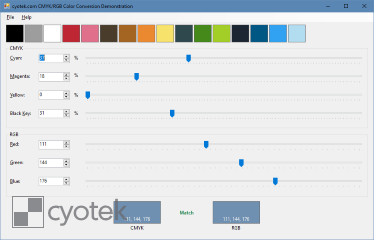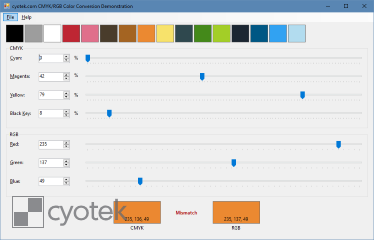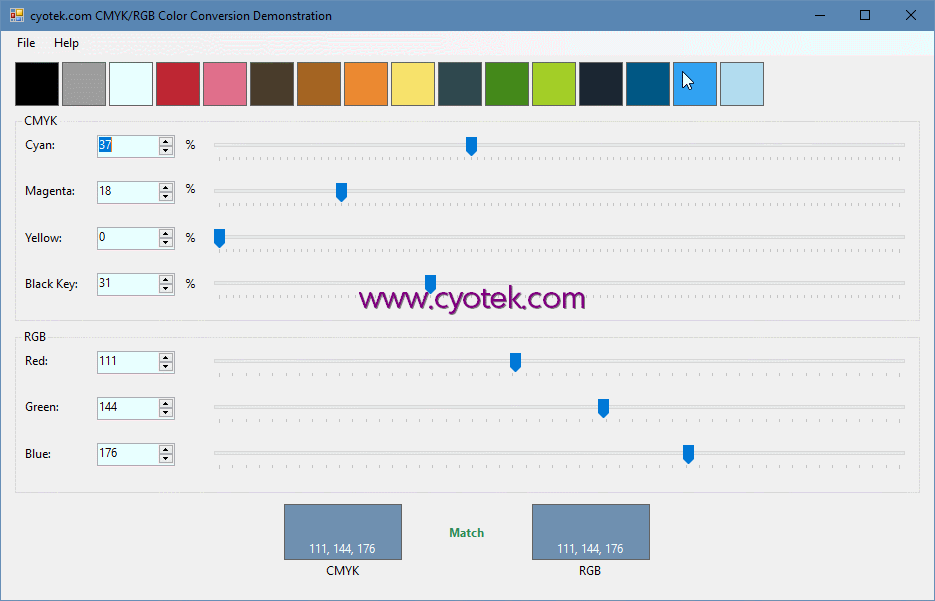## Converting colours between RGB and CMYK in C#

In my previous articles on reading and writing colours from various palette/swatch formats, I left CMYK conversion as an exercise for the reader and only demonstrated RGB aspects. This article demonstrates how to convert colours in CMYK format to RGB and vice versa.Image credit: SharkD [CC0], from Wikimedia CommonsCMYK is traditionally used in the printing industry. It is a subtractive colour model and uses four colours (cyan, magenta, yellow and black). Unlike additive colour models (e.g. the RGB model normally discussed on this blog), combining all primary colours in subtractive results in black, whereas combining all primary colours in additive results in white.

Unlike RGB which generally uses the range `0-255`, most examples of CMYK I've seen use percentages instead, e.g. 37% cyan, 18% magenta, no yellow and 31% black. In this article I'm using the range `0-1` to describe the colours. (Just to be awkward, the demonstration front end uses `0-100` to make it more user friendly!)

### A caveat on conversion

As RGB uses completely different colour channels as well as different value ranges, conversions may not be directly compatible. For example, converting from RGB to CMYK and then back to RGB there is a chance to have a final RGB value that is just slightly off from the source. And that's before we even get into gamut and colour profiles, neither of which I'll be covering in this article.### Converting CMYK to RGB

Red is calculated from the cyan and black colours

R = 255 × (1-C) × (1-K)

Green is calculated from the magenta and black colours

G = 255 × (1-M) × (1-K)

Blue is calculated from the yellow and black colours

B = 255 × (1-Y) × (1-K)

Formula credit: RapidTables

The following function converts CMYK into RGB

``````public static Color ConvertCmykToRgb(float c, float m, float y, float k)
{
int r;
int g;
int b;

r = Convert.ToInt32(255 * (1 - c) * (1 - k));
g = Convert.ToInt32(255 * (1 - m) * (1 - k));
b = Convert.ToInt32(255 * (1 - y) * (1 - k));

return Color.FromArgb(r, g, b);
}``````

### Converting RGB to CMYK

The R,G,B values are divided by 255 to change the range from `0-255` to `0-1`:

R = R/255
G = G/255
B = B/255

The black key is calculated from the maximum red, green or blue colour

K = 1-max(R, G, B)

Cyan is calculated from red and black

C = (1-R-K) / (1-K)

Magenta is calculated from green and black

M = (1-G-K) / (1-K)

Yellow is calculated from blue and black

Y = (1-B-K) / (1-K)

Formula credit: RapidTables

The following functions convert RGB to CYMK

``````public static CmykColor ConvertRgbToCmyk(int r, int g, int b)
{
float c;
float m;
float y;
float k;
float rf;
float gf;
float bf;

rf = r / 255F;
gf = g / 255F;
bf = b / 255F;

k = ClampCmyk(1 - Math.Max(Math.Max(rf, gf), bf));
c = ClampCmyk((1 - rf - k) / (1 - k));
m = ClampCmyk((1 - gf - k) / (1 - k));
y = ClampCmyk((1 - bf - k) / (1 - k));

return new CmykColor(c, m, y, k);
}

private static float ClampCmyk(float value)
{
if (value < 0 || float.IsNaN(value))
{
value = 0;
}

return value;
}``````

You might notice that it is possible for `(1 - k)` to return 0 if `k` is calculated to be solid black (`1`). As this is using floating point math, you don't get the normal `DivideByZeroException`, but instead of the result of the call is `NaN`. The `ClampCmyk` function make sure that values less than zero are normalised as zero, and it also does the same for `NaN` values.

I did write a version of the function that checked to see if `(1 - k)` was `0` and then return zero for the `c`, `m` and `y` fields but the above version was easier to read.

### Demonstration programThe demonstration program lets you freely convert CMYK to RGB and RGB to CMYK uses the above functions, and a lot of sliders and spin buttons. I also included some predefined RGB colours, courtesy of Arne's 16 colour palette.

Filename Description Version Release Date
CmykRgbColorConversion.zip
• sha256: cfc2141a0f0820962621bc35e10882e648c95496751b65ded610a0009faae5a6

Sample project for the converting colours between RGB and CMYK in C# blog post.

15/07/2018

While we appreciate comments from our users, please follow our posting guidelines. Have you tried the Cyotek Forums for support from Cyotek and the community?

Styling with Markdown is supported